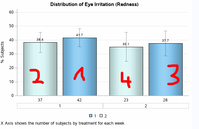## Change bar ordering - sgrender

Hi, its me again,

I have a maybe very easy question:

How do I change the order or my bars in the grouped bar chart of sgrender??

I want it in the swapped order (shown below in red) and not in the order of my x=n.

How can do this?My used code is below.

```proc format;
value xvarf
1 = '1'
2 = '2'
3 = '3'
4 = '4';
value groupf
1 = '1'
2 = '2';
run;

data eyeIrritation;
attrib param length=\$100 label='PARAMETER';
attrib percent length=8 label='Percentage';
attrib time length=3 format=XVARF.;
attrib chartvar length=3 label='Treatment group:' format=GROUPF.;
attrib lcl length=8 label='Lower confidential limit';
attrib ucl length=8 label='Upper confidential limit';
attrib n length=8 label='Number of subjects';
attrib m length=8 label=' of subjects';
infile datalines dsd;
input param percent time chartvar lcl ucl n m;
datalines4;
Beratung, 41.7261905, 1, 1, 35.2002330, 48.2521479, 42, 1
Beratung, 38.3783784, 1, 2,31.0899430,45.6668138,37, 2
Beratung, 37.6785714, 2, 1,28.7006360,46.6565069,28, 3
Beratung, 35.0869565, 2, 2,24.6303204,45.5435926,23, 4

;;;;

title;
proc template;
define style styles.blue;
parent = styles.default;
class GraphColors
"Abstract colors used in graph styles" /
'gdata'  = cxaFb3cF
'gdata3' = cx8CaEdF
'gdata2' = cx5A96DE
'gdata1' = cx42659C;
style color_list from color_list
"Abstract colors used in graph styles" /
'bgA'   = cxe0e7e7;
end;
run;

proc template;
define statgraph EyeIrritation;
begingraph / datacolors=(CX87CEFA CXE0FFFF );
entrytitle 'Distribution of Eye Irritation (Redness)';
entryfootnote halign=left 'X Axis shows the number of subjects by treatment for each week';
layout gridded / border=false;
layout datalattice columnvar=time / headerlabeldisplay=value cellwidthmin=50
columnaxisopts=(display=(line tickvalues))
rowaxisopts=(offsetmin=0 linearopts=(viewmax=60 tickvaluepriority=true )
label='% Subjects' griddisplay=on);
layout prototype / walldisplay=(fill);
barchart x=n y=percent / group=chartvar name='a' barlabel=true skin=modern
outlineattrs=(color=black);
scatterplot x=n y=percent / yerrorlower=lcl yerrorupper=ucl markerattrs=(size=0)
errorbarattrs=(thickness=1.5 color=black) datatransparency=0.6;
endlayout;
endlayout;
entry ' ';
discretelegend 'a' / title=' '  border=false ;
endlayout;
endgraph;
end;
run;

ods listing close;
ods html style=styles.blue image_dpi=100 file='EyeIrritation.html' path='.';
ods graphics / reset noborder width=600px height=400px
imagename='EyeIrritation' imagefmt=gif noscale;

proc sgrender data=eyeIrritation template=EyeIrritation;
format percent 5.1;
run;```
2 REPLIES 2

## Re: Change bar ordering - sgrender

Display order of categorical variables is quite often as the order seen by the graphing procedure. Exceptions would be actual numerical axis variables with an axis that expects numerical order.

So sorting by the group and subgroup variables prior to graphing often addresses such issues. Proc sort does allow descending order mixed with ascending per variable if that helps.DanH_sas
SAS Super FREQ

## Re: Change bar ordering - sgrender

For this plot, the easiest way is to set REVERSE=TRUE in the COLUMNAXISOPTS options.

Discussion stats
• 2 replies
• 161 views
• 1 like
• 3 in conversation